# CH 3 1 SOLVING SYSTEMS USING GRAPHS EQ

• Slides: 13
Download presentation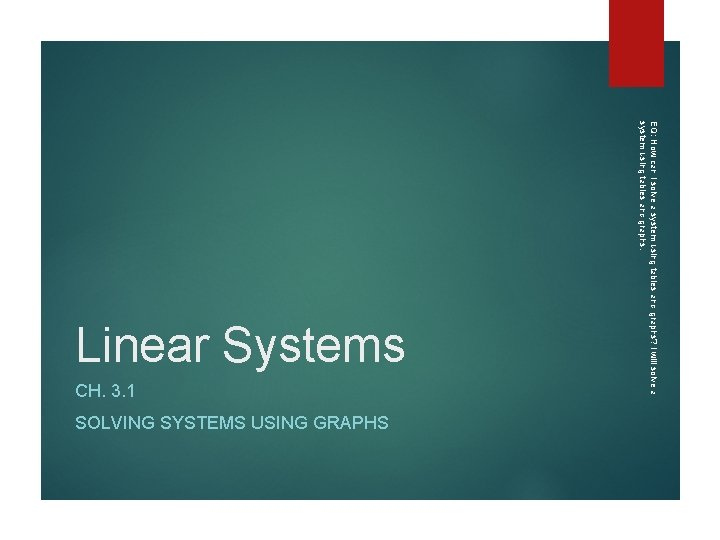CH. 3. 1 SOLVING SYSTEMS USING GRAPHS EQ: How can I solve a system using tables and graphs? I will solve a system using tables and graphs. Linear Systems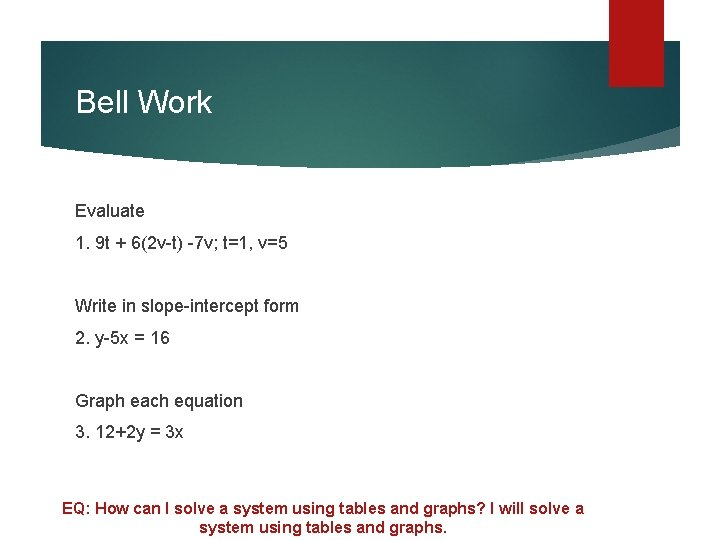Bell Work Evaluate 1. 9 t + 6(2 v-t) -7 v; t=1, v=5 Write in slope-intercept form 2. y-5 x = 16 Graph each equation 3. 12+2 y = 3 x EQ: How can I solve a system using tables and graphs? I will solve a system using tables and graphs.Solving Systems using graphs Linear systems of two equations can be analyzed using the nature of the solutions. One solution 2 lines intersect at one point The slopes of the lines are not equal The y-intersects may or may not be equal Infinitely many solutions 2 lines coincide The slopes are equal The y-intersects are equal No solution 2 parallel lines Same slope, different y-intercept EQ: How can I solve a system using tables and graphs? I will solve a system using tables and graphs.Solving a system by graphing EQ: How can I solve a system using tables and graphs? I will solve a system using tables and graphs.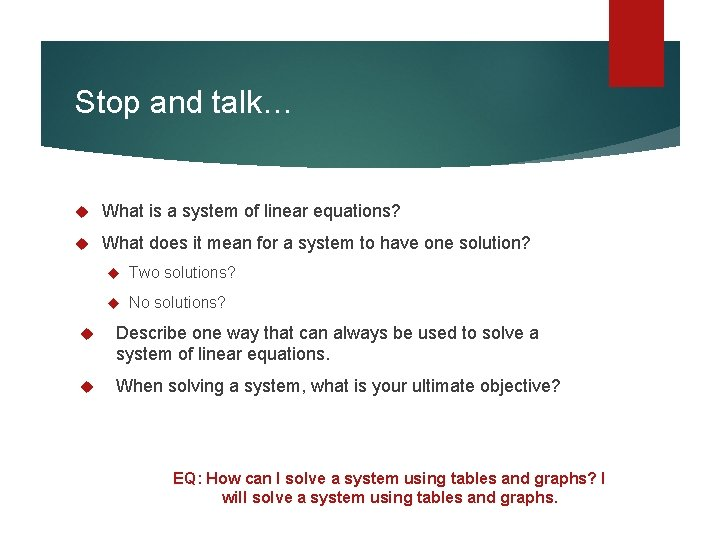Stop and talk… What is a system of linear equations? What does it mean for a system to have one solution? Two solutions? No solutions? Describe one way that can always be used to solve a system of linear equations. When solving a system, what is your ultimate objective? EQ: How can I solve a system using tables and graphs? I will solve a system using tables and graphs.Solving a system by graphing EQ: How can I solve a system using tables and graphs? I will solve a system using tables and graphs.Solving a system by graphing Now you try… 1. 2. EQ: How can I solve a system using tables and graphs? I will solve a system using tables and graphs.Concept Summary EQ: How can I solve a system using tables and graphs? I will solve a system using tables and graphs.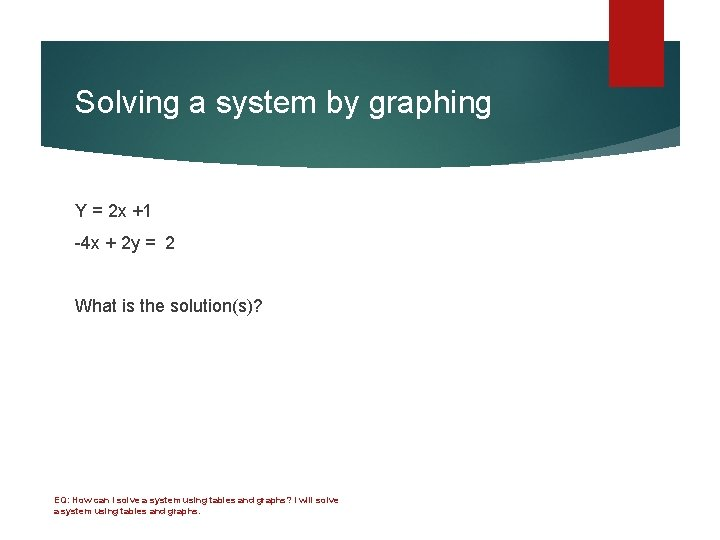Solving a system by graphing Y = 2 x +1 -4 x + 2 y = 2 What is the solution(s)? EQ: How can I solve a system using tables and graphs? I will solve a system using tables and graphs.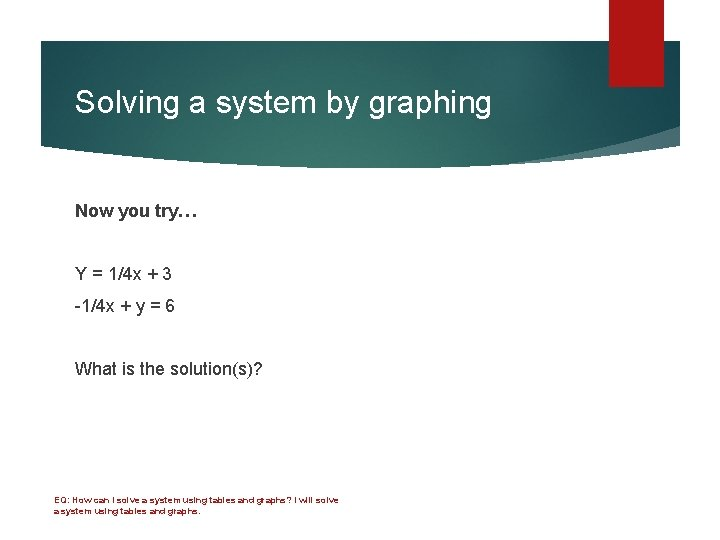Solving a system by graphing Now you try… Y = 1/4 x + 3 -1/4 x + y = 6 What is the solution(s)? EQ: How can I solve a system using tables and graphs? I will solve a system using tables and graphs.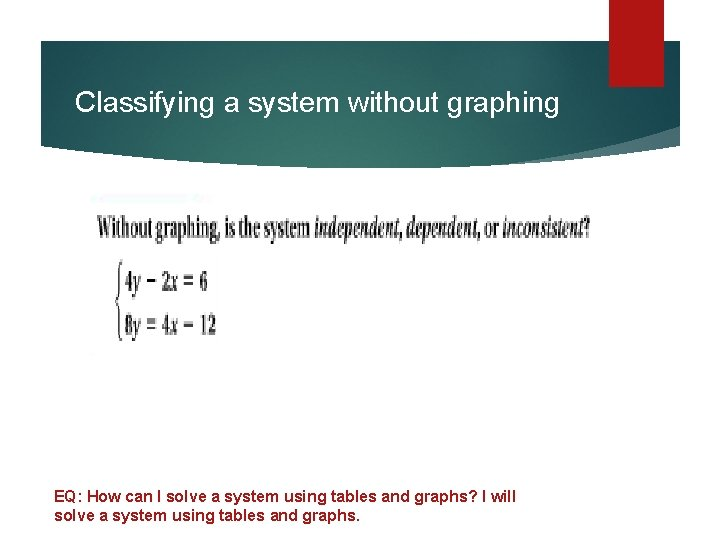Classifying a system without graphing EQ: How can I solve a system using tables and graphs? I will solve a system using tables and graphs.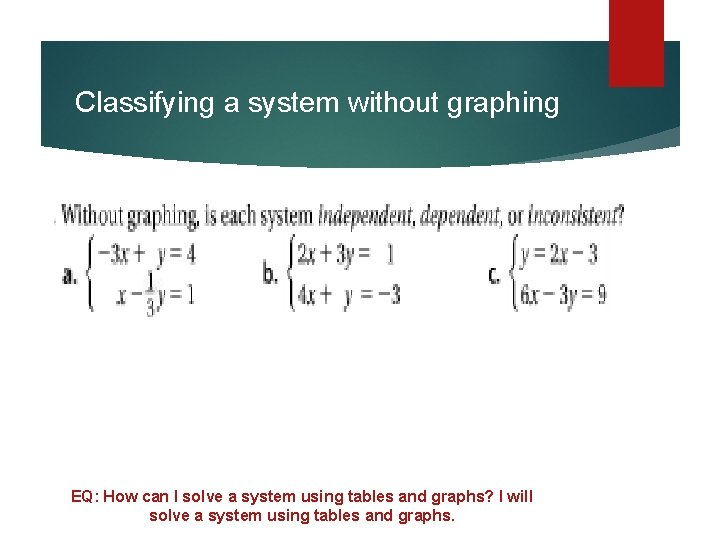Classifying a system without graphing Now you try… EQ: How can I solve a system using tables and graphs? I will solve a system using tables and graphs.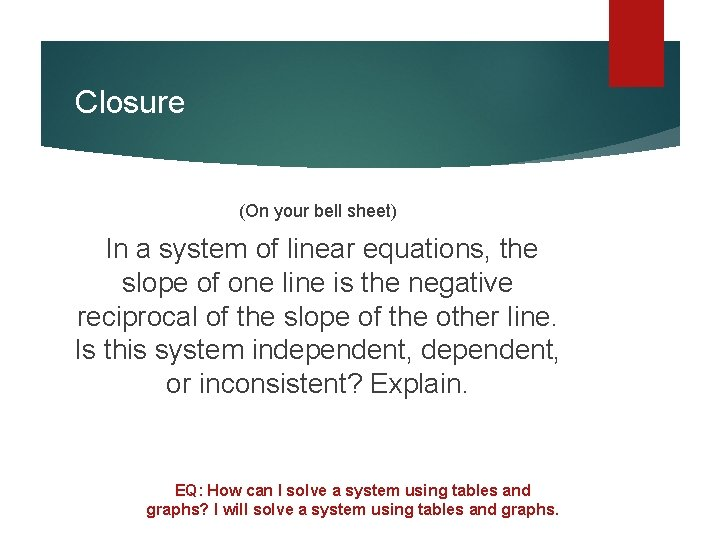Closure (On your bell sheet) In a system of linear equations, the slope of one line is the negative reciprocal of the slope of the other line. Is this system independent, or inconsistent? Explain. EQ: How can I solve a system using tables and graphs? I will solve a system using tables and graphs.